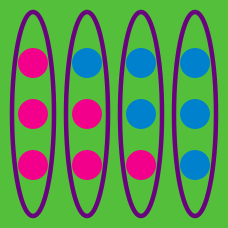Probability

# Combinations - With Restriction

Suppose $5$ distinct numbers are chosen from nine integers $1, 2, \ldots, 9$ to create a $5$-digit number. Given that the digits chosen must consist of $3$ odd numbers and $2$ even numbers, how many distinct $5$-digit numbers can be created?

3 red balls and $12$ blue balls are randomly placed in a row. What is the probability that no two red balls are adjacent?

Suppose a $4$-digit number is created from $4$ distinct integers chosen among $\{1, 2, 3, 4, 5, 7, 9\}.$ How many such $4$-digit numbers include both $1$ and $2$ but neither begin nor end with $1?$

In Sue's Fruit & Flowers Market, there are $8$ different kinds of fruit, including peaches and apples. There are also $6$ different kinds of flowers, including roses and lilies. How many ways are there to make a gift basket such that the basket has $3$ different kinds of fruit including peaches and $2$ different kinds of flowers with no roses?

From a group of $11$ students including $A$ and $B$, $4$ people are selected to be arranged in a line. If both $A$ and $B$ must be among the $4$ selected students, how many distinct line-ups are possible?

×